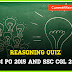## 728x90 AdSpace

Saturday, June 13, 2015

# Reasoning Practice Quiz for SBI PO 2015 and SSC CGL 2015

Friends...Here are some Useful Reasoning Quiz Questions for SBI PO 2015 and SSC CGL 2015. In case of doubt in any questions please comment, we are here to help. Letz discuss and study.

1. Which of the following interchange of signs would make the equation correct?
8 x 6 + 2 = 22
(A) +, x, 2 and 6
(B) +, x, 2 and 8
(C) +, x, 6 and 8
(D) +, x, 2 and 22

2. If ' -'stands for addition, '+' stands for subtraction, '÷' stands for multiplication and 'x' stands for division, then which one of the following equation is correct?
(A) 50 x 5 ÷ 2 - 30 + 25 = 25
(B) 50 - 30 + 5 ÷ 2 x 30 = 25
(C) 40 + 35 x 2 - 50 ÷ 30 = 95
(D) 30 x 2 - 25 + 50 ÷ 5 = 100

Directions : In Question Nos. 3 and 4 some equations are solved on the basis of certain system. Find out the correct answer for the unsolved equation on that basis.
3. 2 x4 x 6 =4; 9 x 3 x7=13; 4x 7x 6=3 ; 9x 7 x 8 =?
(A) 10
(B) 09
(C) 08
(D) 07

4. 3 x5 x 7 x 2=24; 7 x 4 x 6 x8=22, 4 x 4 x 8 x 9=?
(A) 33
(B) 25
(C) 144
(D) 1152

Directions (Q. Nos. 5): Select the related word/letter/numbers from the given alternatives.
5. SOCIAL : OCIALS : : DRIVEN: ?
(A) VENRID
(B) NEVIRD
(C) RIVEND
(D) VWEND

6. Embarrassed : Humiliated : : Frightened : ?
(A) Terrified
(B) Agitated
(C) Courageous
(D) Reckless

7. Macabre : Lovely : : Baneful :?
(A) Unharmful
(B) Owlish
(C) Filter
(D) Ugly

8. AYRRJC : CATTLE : : NCPDCAR : ?
(A) SUBJECT
(B) NEGLECT
(C) PERFECT
(D) OPERATE

In Question Nos. 9 and 10 find the odd word/letters/numbers flow the given alternatives
9. (A) Square
(B) Trapezium
(C) Cylinder
(D) Parallelogram

10. (A) Yacht
(B) Submarine
(C) Boat
(D) Ship
Answers
1) Ans. (C) +, x, 6 and 8
2) Ans. (A) 50 x 5 ÷ 2 - 30 + 25 = 25
3) Ans. (A) 10
4) Ans. (A) 33
5) Ans. (C) RIVEND
6) Ans. (A) Terrified
7) Ans. (A) Unharmful
8) Ans. (C) PERFECT
9) Ans. (C) Cylinder
10) Ans. (B) Submarine

Reasoning Practice Quiz for SBI PO 2015 and SSC CGL 2015Reviewed by Currentaffairs4examz on 13.6.15 Rating: 5 Friends...Here are some Useful Reasoning Quiz Questions for SBI PO 2015 and SSC CGL 2015 . In case of doubt in any questions please commen...